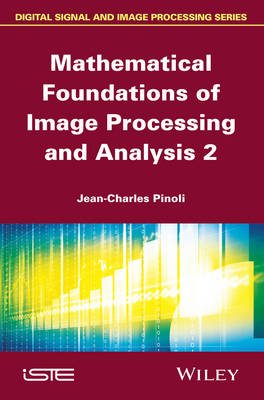•# Mathematical Foundations of Image Processing and Analysis, Volume 2 (Hardback)

(author)
£159.00
Hardback 496 Pages / Published: 04/07/2014
• We can order this

Usually dispatched within 1 week

Mathematical Imaging is currently a rapidly growing field in applied mathematics, with an increasing need for theoretical mathematics.

This book, the second of two volumes, emphasizes the role of mathematics as a rigorous basis for imaging sciences. It provides a comprehensive and convenient overview of the key mathematical concepts, notions, tools and frameworks involved in the various fields of gray-tone and binary image processing and analysis, by proposing a large, but coherent, set of symbols and notations, a complete list of subjects and a detailed bibliography. It establishes a bridge between the pure and applied mathematical disciplines, and the processing and analysis of gray-tone and binary images. It is accessible to readers who have neither extensive mathematical training, nor peer knowledge in Image Processing and Analysis.

It is a self-contained book focusing on the mathematical notions, concepts, operations, structures, and frameworks that are beyond or involved in Image Processing and Analysis. The notations are simplified as far as possible in order to be more explicative and consistent throughout the book and the mathematical aspects are systematically discussed in the image processing and analysis context, through practical examples or concrete illustrations. Conversely, the discussed applicative issues allow the role of mathematics to be highlighted.

Written for a broad audience - students, mathematicians, image processing and analysis specialists, as well as other scientists and practitioners - the author hopes that readers will find their own way of using the book, thus providing a mathematical companion that can help mathematicians become more familiar with image processing and analysis, and likewise, image processing and image analysis scientists, researchers and engineers gain a deeper understanding of mathematical notions and concepts.

Publisher: ISTE Ltd and John Wiley & Sons Inc
ISBN: 9781848217485
Number of pages: 496
Weight: 872 g
Dimensions: 241 x 161 x 32 mm